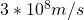# Science Class 8- Chapter 13- Sound- NCERT Exercise solution ( Question-Answer)

Science Class 8 – Chapter 13- sound – NCERT exercise solution (Question-Answer) is provided below. Total 13 Questions are in this NCERT Exercise, all are solved here.

Q1. Sound can travel through

(a) gases only                        (b) solids only

(c) liquids only                        (d) solids, liquids, and gases.

Answer- (d) solids, liquids, and gases.

Q2. Voice of which of the following is likely to have minimum frequency?

(a) Baby girl                           (b) Baby boy

(c) A man                                (d) A women

Q3. In the following statements, tick ‘T’ against those which are true, and ‘F’ against those which are false.

(a) Sound cannot travel in vacuum. (T/F)

(b) The number of oscillations per second of a vibrating object is called its time period. (T/F).

(c) If the amplitude of vibration is large, sound is feeble. (T/F)

(d) For human ears, the audible range is 20 Hz to 20,000 Hz (T/F)

(e) The lower the frequency of vibration, the higher is the pitch(T/F)

(f) Unwanted or unpleasant sound is termed as music. (T/F)

(g) Noise pollution may cause partial hearing impairment. (T/F)

(a) Sound cannot travel in vacuum.        (T)✔️

(b) The number of oscillations per second of a vibrating object is called its time period.            (F)❌

(c) If the amplitude of vibration is large, sound is feeble.          (F)❌

(d) For human ears, the audible range is 20 Hz to 20,000 Hz (T)✔️

(e) The lower the frequency of vibration, the higher is the pitch.      (F)❌

(f) Unwanted or unpleasant sound is termed as music.         (F)❌

(g) Noise pollution may cause partial hearing impairment.      (T)✔️

Q4. Fill in the blanks with suitable words.✍️

(a) Time taken by an object to complete one oscillation is called ____________.

(b) Loudness is determined by the _____________ of vibration.

(c) The unit of frequency is ________.

(d) Unwanted sound is called __________.

(e) Shrillness of a sound is determined by the ________ of vibration.

Q5. A pendulum oscillates 40 times in 4 seconds. Find its time period and frequency.

Number of oscillations = 40

Time taken = 4 sec

Time period = time taken/no. of oscillations = 4/40 = 0.1 sec

Frequency =no. of oscillations/ time taken=  1/time period

= 40/4

= 10 Hz.

Q6. The sound from a mosquito is produced when it vibrates its wings at an average rate of 500 vibrations per second. What is the time period of the vibration?

No. of vibrations per second = 500

Time taken = 1 sec

Time period = time taken/ no. of vibrations

= 1/500 = 0.002 sec.

Q7. Identify the part which vibrates to produce sound in the following instruments.

(a) Dholak

(b) Sitar

(c) Flute

Q8. What is the difference between noise and music? Can music become noise sometimes?

Yes, music can become noise sometimes when it becomes too loud making it unpleasant to the ear. Hence, unpleasant sound to the ear is called noise.

Q9. List sources of noise pollution in your surroundings.

Answer-  List of sources of noise pollution in my surrounding

• Traffic ( sounds of vehicles)
• Sound of loudspeakers, TV, etc.
• Sound of bursting crackers.
•  Sound of electric generators
• Home appliances such as mixer, cooler, etc.

Q10. Explain in what way noise pollution is harmful to human.

Answer- Noise pollution can lead the number of health problems such as-

• Hearing loss
• Hypertension
• Stress
• etc.

Answer- I will suggest my parent to buy a house which is three lanes away from the roadside because, on the roadside, there is too much noise due to the sound of running vehicles. But in three-lane away from the roadside, the intensity of noise decreases.

Q12. Sketch larynx and explain its function in your own words.

Functions of the larynx:

The larynx is also called a voice box. Larynx moves when we swallow something. There were two vocal cords are stretched across the voice box in such a way that it leaves a narrow slit between them for passage of air.

When we speak, the air is formed into this gap by lungs which prompt vocal cords to vibrate.

Since, vibrating object produce sounds, muscles attached to vocal cords can make the cord tight or loose. When the vocal cords are tight, the quality of voice is different from that when they are loose.

Q13. Lightning and thunder take place in the sky at the same time and at the same distance from us. Lightning is seen earlier and thunder is heard later. Can you explain why?

Answer- Because the speed of light is more than the speed of sound. So, lightning is seen earlier than thunder is heard later.

Speed of sound= 343 m/s 0r 2.9 km/s may be.

Speed of light= 299792458 m/s

=Science Class 8 chapter 13 – sound – NCERT Exercise Solutions ( Question-Answer) Ended. 👍👍👍

error: